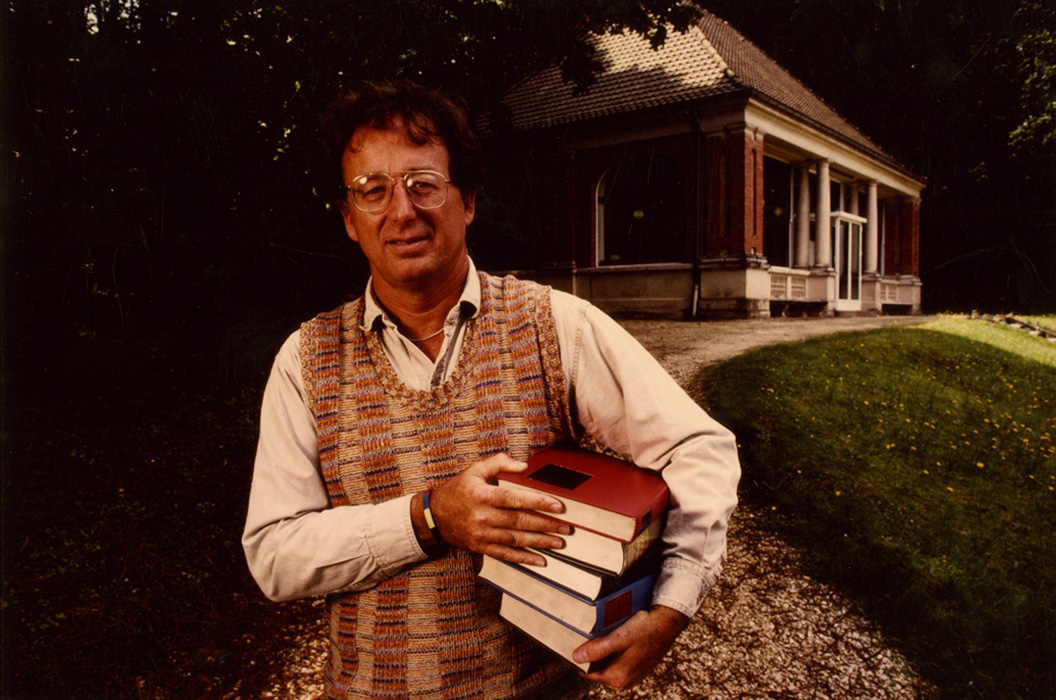# Localization, Periodicity, and Galois Symmetry (Spring 2023)

Sullivan introduced the idea of localizing and completing spaces at primes, which led to important advances in topology and homotopy theory. For example, it allowed natural actions of the Galois groups on objects associated to manifolds to be studied in relation with periodicity theorems. One concrete result of this theory is the proof of the Adams conjecture using etale homotopy. We will follow Sullivan's notes [S2] in this seminar. Aside from some algebraic topology which we will spend some time reviewing, these notes build this theory essentially from scratch.

#### Schedule

Jan 30
Caleb Ji
Organizational meeting, Algebraic constructions
We will explain the plan for this seminar and begin Chapter 1 of [S2]. We will spend some extra time reviewing sme topological background, including equivariant cohomology and K-theory.
Feb 6
Caleb Ji
Homotopy theoretical localization
We will define localization of topological spaces and construct it. Then we will give several examples. This follows Chapter 2 of [S2].
Feb 13
Caleb Ji
Homotopy theoretical completion I
We will define completion of topological spaces in the homotopy category as a functor and show it is representable in many cases using Brown representability. We will then give some examples and compute some of the homotopy groups and cohomology groups of the completion. This follows Section 3 of [S1].
Feb 20
Caleb Ji
Homotopy theoretical completion II
We will begin by defining good homotopy groups and continue the calculation of the homotopy groups of the completion. Then we will define l-finite and formal completion. This will allow us the describe the genetics of a homotopy type, which essentially allows us to reconstruct it from its rational and p-adic pieces. This follows Section 3 of [S1].
Feb 27
Caleb Ji
Etale homotopy I
We will construct the etale homotopy type of a scheme, which is a pro-homotopy type which recovers etale cohomology. We will also discuss some variants involving rigidification and completion which refine the etale homotopy type.
Mar 6
No meeting due to the AWS
Mar 13
Spring break
Mar 20
Kevin Chang
Etale homotopy theory of classifying spaces and finite Chevalley groups
We will cover the computation of the cohomology of GL_n(F_q), U_n(F_q), SO_n(F_q), and more. The key ingredients are the comparison theorem for classifying spaces and the cohomological Lang fiber square, which relates the classifying spaces of finite Chevalley groups to the classifying spaces of the corresponding groups over F_q bar.
Mar 27
Caleb Ji
We will introduce spherical fibrations and Adams operations in order to state the Adams conjecture. Then we will give an application to computing the image of the J-homomorphism.
Apr 3
Caleb Ji
We will outline Sullivan's proof of the Adams conjecture. A key ingredient is the action of the absolute Galois group on etale homotopy types.
Apr 10
Caleb Ji
The Beilinson conjectures I
We will review some basic material on zeta functions and give an introduction to Deligne cohomology. This leads to the definition of higher regulators, which can be used to state Beilinson's conjectures.
Apr 17
Caleb Ji
The Beilinson conjectures II
We will introduce the absolute cohomology groups of Beilinson, which are defined using algebraic K-theory. These give rise to Chern class maps into Deligne cohomology, which allow us to state Beilinson's conjectures.
Apr 24
Caleb Ji
Etale Steenrod operations and the Artin-Tate pairing I
The Artin-Tate pairing is a pairing on the torsion of the Brauer group of a surface, which Tate conjectured to be alternating. We will begin an exposition of Tony Feng's proof of this conjecture in this talk. We will explain the background to this problem and construct Steenrod squares and Bockstein operations in etale cohomology which will be used in the proof.
May 1
Caleb Ji
Etale Steenrod operations and the Artin-Tate pairing II
We begin by describing Stiefel-Whitney classes in \'etale cohomology. Then we prove a Wu theorem in \'etale cohomology, which makes use of relative \'etale homotopy theory. This allows us to show that the obstruction for the Artin-Tate pairing to being alternating vanishes, completing Feng's proof of Tate's conjecture.